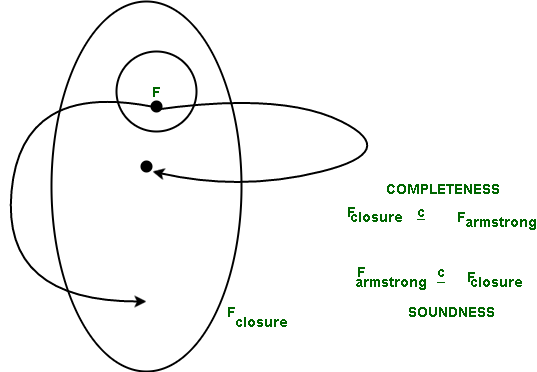Related Articles

# Proving Soundness of Armstrong’s Axioms

• Difficulty Level : Expert
• Last Updated : 21 Jun, 2020

Prerequisite – Armstrong’s Axioms in Functional Dependency in DBMS
Armstrong mentioned that rules 1 through 3 have completeness along with soundness. Armstrong axioms are sound as they do not generate any incorrect Functional Dependencies and it allows us to generate the F+ closure.

The proof of Soundness –
Set of functional dependencies that can be derived with logic from a given set of functional dependencies (Fclosure) and the set of functional dependencies that can be inferred from Armstrong’s Axioms (Farmstrong) are same. In terms of sets, if we want to show equality, then we mention that one is a proper subset of the other. Hence, we need to prove that,

 Farmstrong  is a proper subset of Fclosure
for soundness.


This means that all things derived from F after applying Armstrong’s Axioms are correct functional dependencies and this property is called Soundness.Consideration for Soundness –has been derived from F using Armstrong’s Axioms after m number of steps.

In each step we would apply the rules of reflexivity, augmentation and transitivity.
(If we derive from Axioms which are only 3 in number, the repeated application of them would also give similar results.)

Rule of Reflexivity –
This rule states that if E is a proper subset of G, then G implies E (). This rule always results in correct Functional Dependencies because it is a trivial functional dependency.

Rrule of Augmentation –
This rule states that for, if we add Z on both sides, then we would get, which would be a correct functional dependency.
If ta and tb have the same corresponding values in G and Z (GZ),
It would mean that they have same corresponding value for G,
which would mean that they have same corresponding value for E, since G implies E,
it would also mean that they correspond to E and Z (EZ).

Rule of Transitivity –
This rule states that if,then G determines Z ()
If ta and tb have the same corresponding values in G
which would mean that they have same corresponding value for E, since G implies E holds,
it would also mean that they correspond to E, since E implies Z holds.

So, applying these 3 rules will always give rise to correct functional dependencies (even if we apply them m number of times).
Hence proved.

Attention reader! Don’t stop learning now. Get hold of all the important CS Theory concepts for SDE interviews with the CS Theory Course at a student-friendly price and become industry ready.

My Personal Notes arrow_drop_up